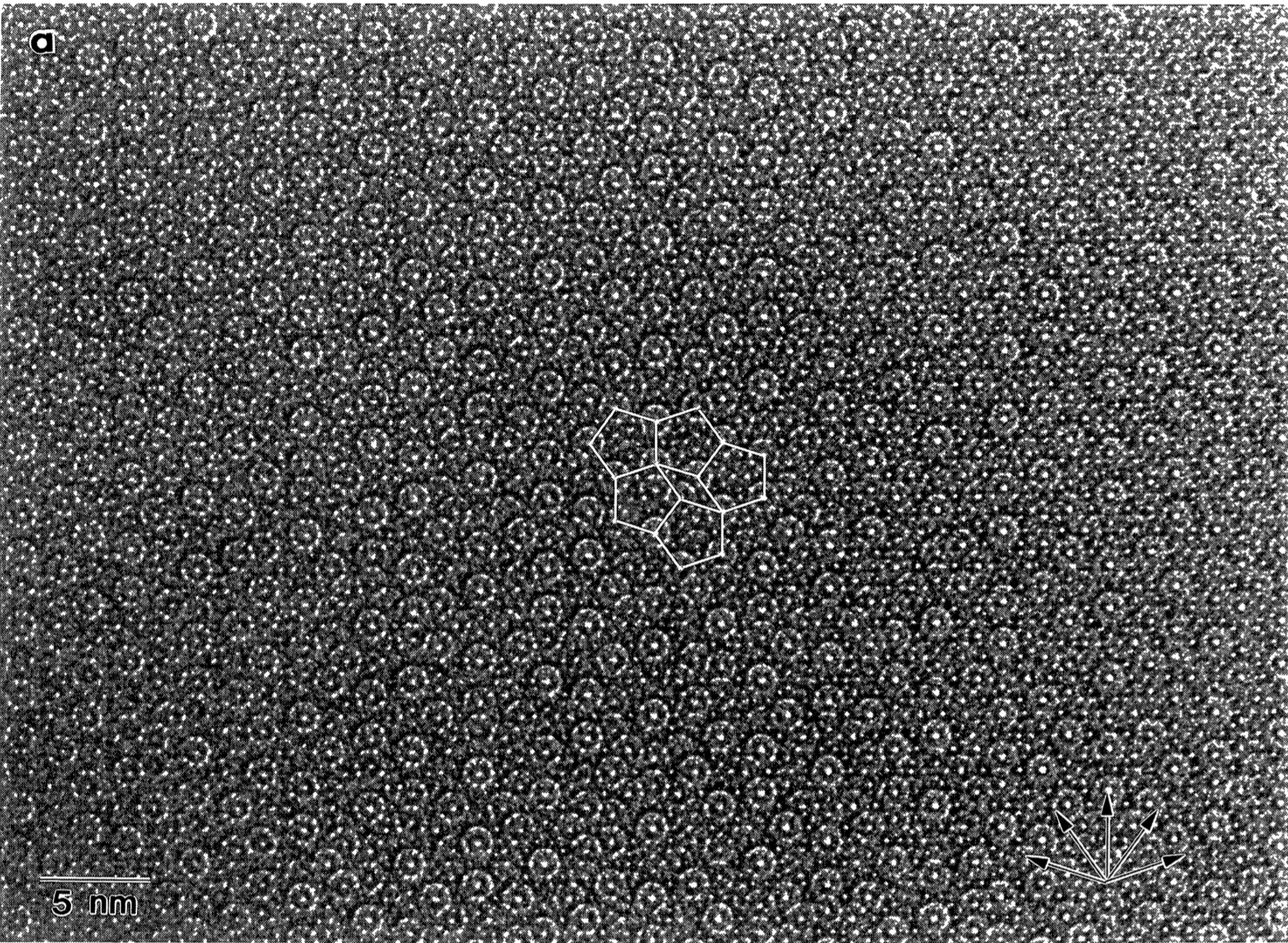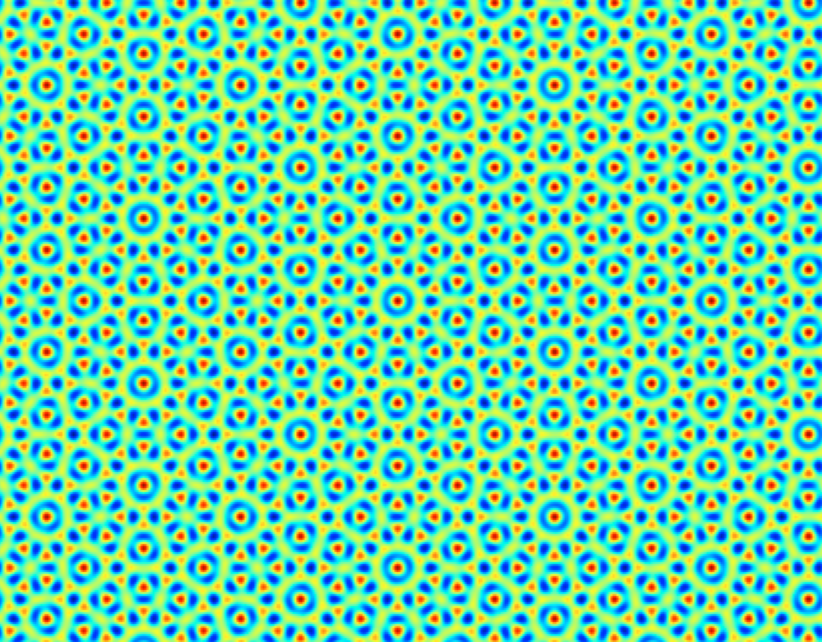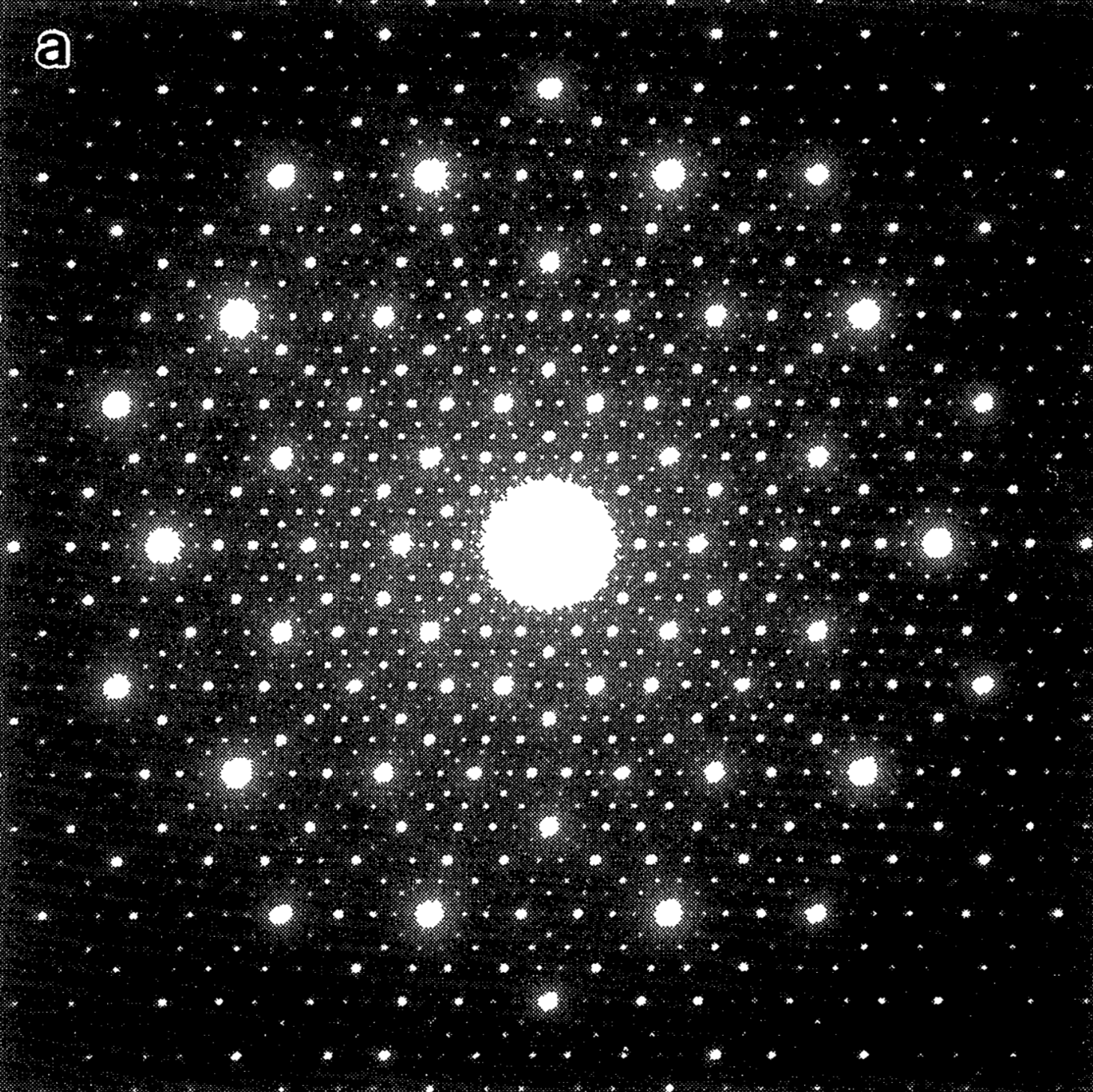# How to Think About Quasicrystals

31 Mar 2018
Quasicrystals, Fourier Space, and Symmetry

I recently gave a journal club talk at the CMX Journal Club about quasicrystals, which happily gave me cover to finally research what the heck they are. Previously, I had a lot of trouble getting my head around the oft-repeated mantra that quasicrystals have long range order, but no translational symmetry. What exactly does this mean, and how is it possible? How do we square it with the fact that quasicrystals can have discrete rotational symmetries?. The answer turns out to be that all these pieces fit together nicely in Fourier space. This viewpoint clarifies and unifies these concepts and helps explain some of the other strange properties of quasicrystals to boot.

## What do They Look Like?

To get on the same page, let’s start by looking at a quasicrystal. To simplify things a bit, I’ll use decagonal AlNiCo quasicrystals as an example. These quasicrystals are crystalline in one dimension and quasicrystalline in the other two, which makes them much easier to visualize than icosahedral quasicrystals. To be precise, these quasicrystals are constructed from repeating layers of atoms along the periodic axis, where each individual layer of atoms is a 2D quasicrystal. Single quasicrystals of this type look more or less like the example below:

Essentially, nothing about its morphology is different from a generic crystal of any metal. It’s shiny, and it has well-formed facets. The only odd part about this crystal is the tenfold, decagonal symmetry! Normal crystals are made up of repeating structures, which is what leads to the regularity and smoothness of their facets. Yet it’s also well known that this kind of repeating structure simply cannot have a rotational symetry axis with higher than sixfold symmetry.

Seeing a tenfold symmetry in the crystal habit tells us immediately that something funky is going on. But what is it? The best way to find out is to look a bit closer, and see where the atoms are.STEM data from a thin section of a decagonal Al-Ni-Co quasicrystal collected by Hiraga et al.

This STEM image is looking along the tenfold axis of a similar decagonal quasicrystal. It’s showing us where the columns of atoms are in the material. In reality the resolution of this image is a bit worse than atomic, but we can clearly identify the repeating structural motifs.

Take a look at these motifs, which look like a ring with a dot in the center. They are scattered around the image in what looks at first like a random way. This randomness is most clearly encapsulated by noting that there is no translation we can apply to the image that brings the whole pattern back to itself. Say it out loud: the quasicrystal has no translational symmetry. This is definitely a good sign, because the incompatibility of tenfold rotational symmetry and translational symmetry is a mathematical fact. I, for one, would rather not break mathematics if I don’t have to.

Yet in this image we can also see the seeds of long range order. The structural motifs fit neatly into vertical lines. These lines extend all the way from the top to the bottom of the image. In fact, there are many different directions along which the motifs line up. These lines don’t just extend across the field of view of this image, in the absence of defects they actually extend all the way from one side of the crystal straight through to the other!

We can’t formalize this just yet, but this is at the heart of what we mean when we say that quasicrystals have long range order. Even though we couldn’t generate the structure by copying a single unit cell, we still find that the final structure has perfect lattice planes running through it, just like we any normal crystal. It’s the existence of these planes that leads to the smooth facets of quasicrystals and to all the properties of quasicrystals that we associate with long range ordering.

The final thing to discuss is the rotational symmetry of the quasicrystal. It’s plain to see that this arrangement of atoms has somehing like tenfold rotational symmetry. For example, if we were to collect a set of all the directions that lattice planes run along, this set of directions would have tenfold rotational symmetry. Yet it’s just as plain to see that there are no actual centers of rotational symmetry in the quasicrystal. So we can clarify our question about rotational symmetry to: how can we formalize the kind of rotational symmetry that a quasicrystal has?

## Mathematical Description

The first question to address, from which everything else follows, is: how can such a structure possibly be mathematically consistent? Consider that any real crystal can be described by various properties that are functions of space - the electron density, the ionic potential, and so on. Each of these mathematical functions should have the same symmetry properties as the pattern of atoms they live in. Our first challenge is therefore to figure out how to write down a mathematical function that has these properties in the first place!

As a toy model for a 2D quasicrystal, consider the function:

$f(\vec{x}) = \sum_{n=1}^{10} \exp(i \vec{x} \cdot \mathbb{R}_n(\hat{i}))$

Where $$\mathbb{R}_n$$ represents a rotation through $$\frac{n \pi}{5}$$. In Fourier space, the function looks like a set of ten $$\delta$$ functions:The structure of this function - a tenfold symmetric pattern of delta functions - shown in Fourier space.

We can visualize this function in real space as well:A section of the real space structure that corresponds to a tenfold symmetric pattern of delta functions in Fourier space.

Surprisingly, it reproduces the essential features of the HRTEM image. It:

• Has repeated structural motifs
• But no overall translational symmetry
• Perfect planes that extend throughout the entire domain
• Tenfold rotational symmetry in the orientations of those planes
• But no true centers of rotational symmetry1

What’s more, this function has a structure in Fourier space that looks a lot like the structure that arises in the functions that describe the properties of normal crystals. As a reminder, in a normal crystal, we expect the Fourier space representation of properties like the electron density to be localized into a series of $$\delta$$ functions lying on a discrete reciprocal lattice.

We seem to be drawn to a description that relates the long range order present in both crystals and quasicrystals to the fact that in Fourier space, they are represented by a set of delta functions. We also can see emerging a relationship between the macroscopic symmetry properties of crystals and quasicrystals and the symmetries in the locations of those delta functions.

## The View from Fourier Space

With this in mind, it’s time to unify our concept of a crystal and a quasicrystal and finally formalize these ideas. Recall that any function describing the spatial variation of a property of a regular crystal can be expanded in a Fourier series of the form

$f(\vec{x}) = \sum_{\{a_i\}} f_{\{a_i\}} \exp(\vec{x} \cdot \sum_{i=1}^d a_i\vec{b}_i)$

Where the set of integer-valued coefficients $$\{a_i\}$$ run from $$1$$ to the dimension of space, and index points on the reciprocal lattice of the crystal. We generalize this definition and declare that all the spatially varying properties of a generic (quasi)crystal can be expanded in a Fourier series of the form

$f(\vec{x}) = \sum_{\{a_i\}} f_{\{a_i\}} \exp(\vec{x} \cdot \sum_{i=1}^n a_i\vec{b}_i)$

Where now the coefficients $$\{a_i\}$$ run from $$1$$ to $$n$$, some number potentially larger than $$d$$. We can see immediately that this is true for the function described in our toy model, but including the full reciprocal lattice gives us many more degrees of freedom to play with. For example, you can notice that our toy model contains lots of little maxima which attain values distributed smoothly over some range. If we wanted to use such a function to describe, for example, the ionic potential associated with a quasicrystal made up of real atoms, we would expect to find only a handful of different kinds of sites. Instead, we see a a smooth continuum of many kinds of sites. Including the full reciprocal lattice will, among other things, let us capture this kind of discreteness in the structure of a real quasicrystal.

There are some complications that arise from allowing $$n$$ to be larger than $$d$$, which I will address quickly here. First, such a description generates lattice vectors that lie arbitrarily close to one another. This would be a problem if we were trying to describe a lattice in real space - say, a lattice of atoms - but it turns out it’s totally fine in reciprocal space. In fact, this property of lattice vectors lying arbitrarily close together is a universal property of quasicrystals, which we can see most clearly in diffraction patterns like the one below.Electron diffraction along the tenfold symmetry axis in an Al-Ni-Co quasicrystal, collected by Hiraga et al.

Our inner pedant might have a further objection. Mathematically, the lattice generated this way (sometimes called the Fourier module) is dense in Fourier space. What is special about these quasicrystalline functions if we’re really just allowing them to take on any value at any point in Fourier space? We can proceed by analogy to a regular crystal. In a crystal, the Fourier components converge to $$0$$ as the coeffients $$\{a_i\}$$ go to $$\infty$$. In a quasicrystal, we will require the same thing - that essentially all the spectral weight in these functions is located at points with small coefficients $$\{a_i\}$$. This additional requirement leads us to expect the kind of pattern we saw above, with most of the spectral weight concentrated on a small subset of peaks in the reciprocal lattice.

Now that we have a valid mathematical description of (quasi)crystals, we can define exactly what we mean by long range order.

• Any object whose properties can be represented by a countable set of delta functions in Fourier space has long range order.

This connects directly to our initial observation that, even though quasicrystals have no translational symmetries, there exist perfect planes of atoms extending through the entire quasicrystal. The concept of perfect planes in real space maps directly onto the existence of sharply localized peaks in Fourier space.

We can also formalize the concept of macroscopic rotational symmetry. If, for any spatially dependent property of an object, the amplitude of that property’s representation in Fourier space respects a particular rotational symmetry, than the object can be said to (macroscopically) have that rotational symmetry.2

Now, I will note that this concept of rotational symmetry can be expanded quite a bit further, and it is even possible to define space groups for quasicrystals based on these ideas, by including the phases of the Fourier components of an object’s properties. I recommend David Mermin’s (Quasi) Crystallography is Better in Fourier Space to the interested reader, it is a delightful polemical which explores the consequences of these definitions further.

## Implications

There are a few implications that emerge from this viewpoint:

• The potential existence of spectral weifght of the ionic potential and electron density at arbitrarily low frequencies implies that we should worry about localization of electrons.
• The structure of the reciprocal lattice doesn’t generate Brillouin zones, instead it completely shreds the dispersion relations and the electronic Fermi surface.
• As a result, we can expect transport properties (thermal and electrical) to be dramatically different from those in regular crystals. This turns out to be the case.
• Defects can exist in quasicrystals just like in regular crystals. For some reason the community has chosen to add to the semantic overloading of the word “phase” and call them “phason defects.”
• Quasicrystals are intrinsically related to incommensurately modulated crystals, with which they share the same mathematical description and many properties.

## Footnotes

1. In fact, it turns out that this particular function has a single center of tenfold symmetry at $$x=0$$. However, the probability that any randomly selected finite volume of the infinite function would contain that center of symmetry is exactly zero. This stands in contrast with a generic symmorphic crystal, which contains a finite density of centers of symmetry.

2. It’s worth considering an analogy with non-symmorphic crystals, which just like quasicrystals have macroscopic rotational symmetries but no exact centers of symmetry.